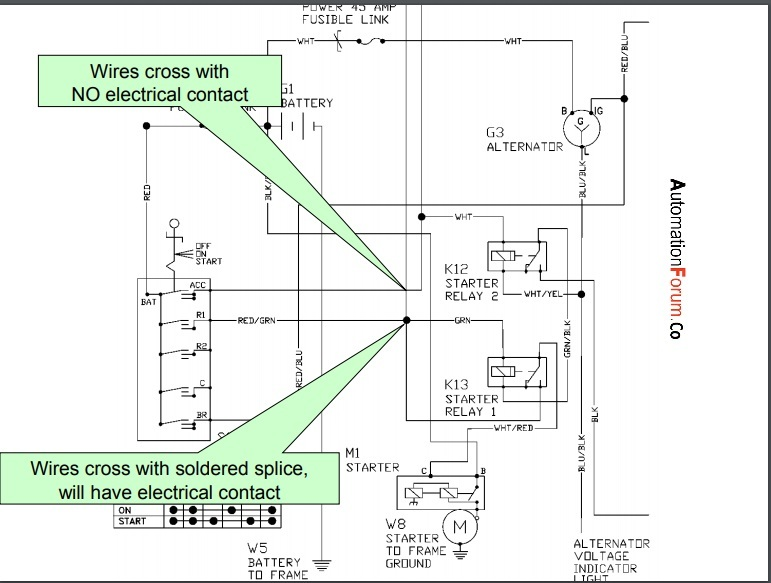# How to read an electrical diagramIn order to understand an electrical diagram, we must know the electrical symbols, an electrical diagram is composed of symbols, words, numbers, and lines. The electrical symbol is used to describe all the installations in an electrical diagram. An electrical diagram describes the flow of electricity or it can also be explained as the diagram that shows the path of electrical current. The flow or path of the electric current is from positive to negative. The flow of electric current will reach the load in an electrical diagram the load is a device that requires electrical power to do its operation it could be a motor or electric bulb etc. Basically an electrical diagram is a diagram that shows the wiring between the components and where the components are exactly located.

#### Why do we need an electrical diagram and what are the uses of an electrical diagram

• The need for an electrical diagram is that we will able to troubleshoot problems by referring to the electrical diagrams
• We can also check if the connections are correct
• We can determine the wiring between the components and the relative position of the components
• We can determine the cause of the problem by checking the electrical diagram

We must consider certain things while reading an electrical diagram at first we must find the load in the diagram after that check for the fuse that provides the power and after that, we must check for the ground source. While looking at the electrical diagram we need to find the ground source and power source and both the power and the ground will be connected to the load in the diagram. In an electrical diagram, the power source will always be given a red color. The power source can be mostly seen at the top of the diagram, we can see the power will travel in the diagram until it finds an open, the ground can always be seen at the bottom of the diagram and it goes upward in the diagram and this will also stop in an open

Another important point that must be known that there won’t be any voltage and current in an open circuit. The color of the ground will be green, if we see orange color in the electrical diagram it means that the device or the wire that is in orange color would only have power if it is switched on. In an electrical diagram, we can see a yellow-colored connection, and the yellow color is used for the connections that would be grounded only if it is switched on. In certain case, we can see a dotted line in the electrical diagram which means that it has more connections than what we see in the image or diagram and this dotted line boxes is the control device. We can also see the solid line boxes or boxes which are not dotted and they are also control devices but its connection or pin is fully shown in the diagram

Scroll to Top